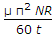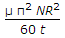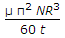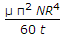# Mechanical Engineering - Hydraulics and Fluid Mechanics

### Exercise :: Hydraulics and Fluid Mechanics - Section 5

36.

The power transmitted through the nozzle is maximum when the head lost due to friction in the pipe is __________ of the total supply head.

 A. one-half B. one-third C. two-third

Answer: Option B

Explanation:

No answer description available for this question. Let us discuss.

37.

In case of flow through parallel pipes,

 A. the head loss for all the pipes is same B. the total discharge is equal to the sum of discharges in the various pipes C. the total head loss is the sum of head losses in the various pipes D. Both (A) and (B)

Answer: Option D

Explanation:

No answer description available for this question. Let us discuss.

38.

The flow at critical depth in an open channel is called torrential flow.

 A. Yes B. No

Answer: Option B

Explanation:

No answer description available for this question. Let us discuss.

39.

The torque required to overcome viscous resistance of a footstep bearing is (where μ = Viscosity of the oil, N = Speed of the shaft, R = Radius of the shaft, and t = Thickness of the oil film)

 A.B.C.D.Answer: Option D

Explanation:

No answer description available for this question. Let us discuss.

40.

Coefficient of velocity is defined as the ratio of

 A. actual velocity of jet at vena contracta to the theoretical velocity B. area of jet at vena contracta to the area of orifice C. actual discharge through an orifice to the theoretical discharge D. none of the above

Answer: Option A

Explanation:

No answer description available for this question. Let us discuss.

#### Current Affairs 2021

Interview Questions and Answers